# Reference Planes

New for December 2005!

S-parameters for a device (in particular their angles), refer to fixed reference planes at each port, whether you are dealing with measured or simulated data. Quite often, the desired reverence plane is NOT where you are able to measure the device, because the device must be mounted in a test fixture in order to measure it. If you know the approximate length of the test fixture you can perform a "poor-man's de-embedding" procedure to move the reference plane of the measurement toward the device. You can also move reference planes just to flatten out phase characteristics, which is particularly useful when looking at phase tracking between two or more widgets.

Below we'll discuss an example where we move the reference planes using Agilent's Eagleware Genesys software, then we'll repeat the same data manipulation using our S-parameter Utilities file which runs in Excel. It will perform the same operation on any device that you have S-parameters for.

We started by downloading the S-parameters of a Hittite amplifier, the HMC397. Hittite is perhaps the only MMIC vendor that makes it easy to download S-parameters on their web site, so that's why we pick on them. They should take it as a compliment!

In Genesys we pull up the S-parameters in an "S2P" block. On the input and output we have added adjustable delays for moving the reference planes. In the initial analysis we have zeroed out the delays so you can look at the raw phase angles.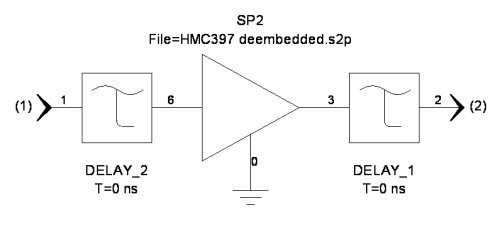Below is the gain magnitude and phase. Not that the phase has a negative slope, which is true of most real devices. This amplifier is DC coupled, so it has gain down to zero Hertz. The slight phase slope is indicative of a part that was RF probed, with the reference plane right at the probe tips.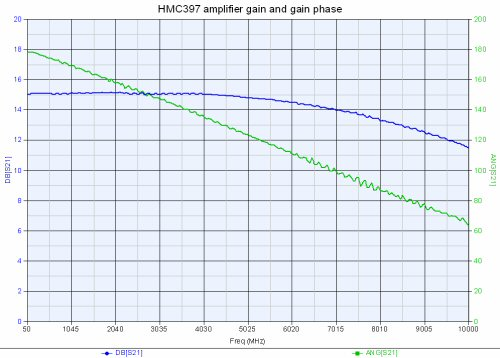Here's the reflection coefficients (S11 and S22) plotted on a Smith chart. You can see that they too have a negative phase slope, as they wind clockwise around the chart, starting at 0 degrees.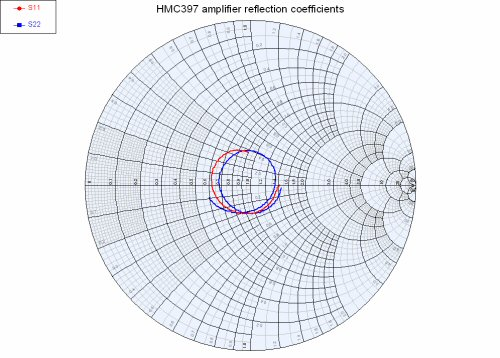Now let's see what happens when we add 0.2 nanoseconds of delay on the input and output. This is the equivalent of moving the reference planes by 6 centimeters away from the device (recall that electromagnetic energy moves almost exactly 30 centimeters in one nanosecond, it's a Microwaves101 rule of thumb). Here is the revised schematic: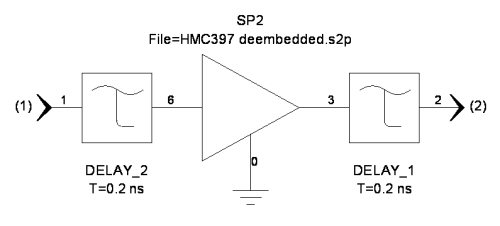Now lets look the gain phase angle. The angle wraps around zero four times. This is more like what you'd see if you measured the device embedded in a small test fixture (perhaps one inch alumina fixture).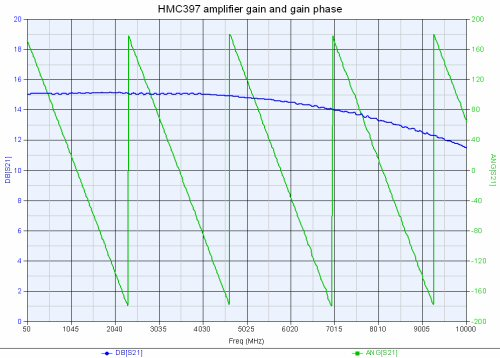Let's also look at the reflection coefficients. They too are now wrapped around the chart multiple times.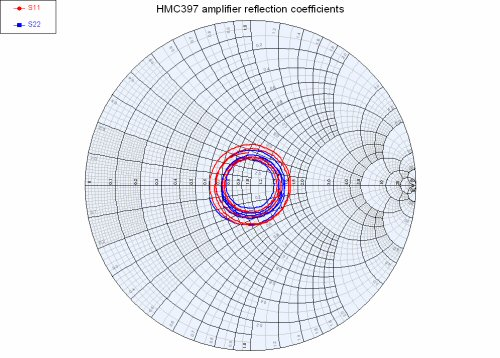Now you can visualize what moving the reference planes does to phase angles of the S-parameters. This example is actually the reverse of how you would move reference plans in real life; you'd take fixtured data that wraps multiple times, subtract delay from it to get "de-embedded" data. The problem with de-embedding this way is that you'll need at least one standalone measurement of the fixture with nothing in it to compute how long it is.

Next we loaded the same HMC397 S-parameters into our S-Parameter Utilities spreadsheet, and made the same gain magnitude/phase plot. It agrees with the first Genesys plot as it should. Unfortunately we don't have Smith chart capability in the spreadsheet, but rest assured that the polar coordinates of the reflection coefficients are correct.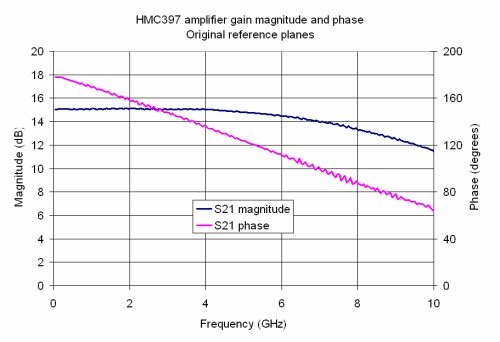Finally, we added -6 centimeters to the input and output reference planes and replotted that gain magnitude and phase. Why -6 and not +6? Call it a Microwaves101 convention, but we believe that "positive" reference plane extensions move into the device, not away from it. The result is the same, our free download performs the same function as a \$5000 piece of linear analysis software, and we won't come after you every year for "maintenance fees!"!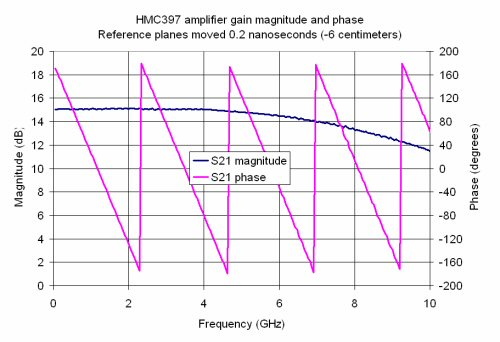What happens to group delay when you move the reference planes? It changes by the amount of delay that you added. Below is the raw data group delay plot. Without moving the reference planes, we see that the device "measures" about 0.04 nanoseconds in length.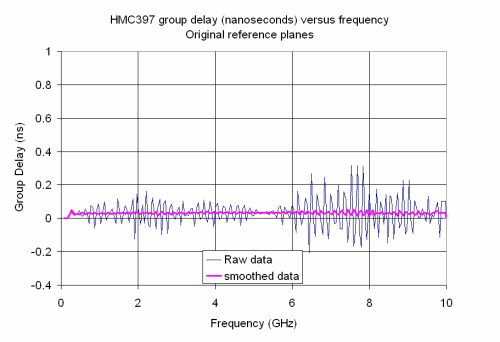Adding 0.2 nanoseconds delay to both the input and the output increases the group delay by 0.4 nanoseconds total, as it should.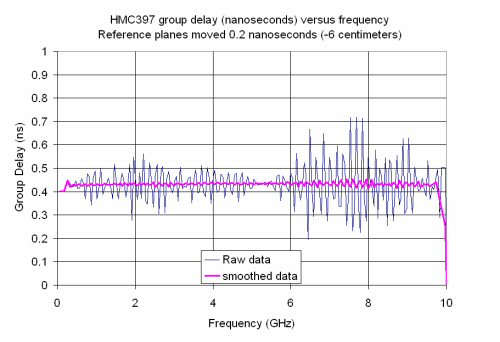### Unwrapped phase

Now let's go back to the original reference planes and plot the "unwrapped" phase of S21 (another option in the S-Parameter Utilities spreadsheet). By unwrapped, we mean that whenever it crosses -180/180 on the Smith chart, we'll fix it so it just keeps going in a straight line. Here it is (although in this case the part has so little phase variation that unwrapping was not needed!):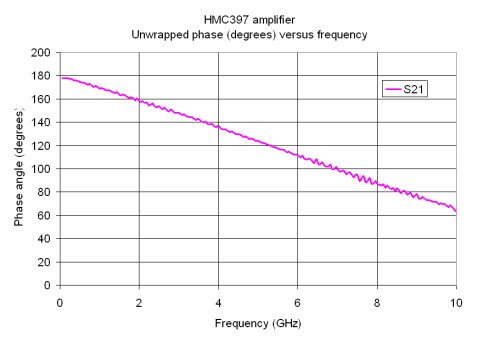You can see some noise in the data, but it is hard to quantify at this scale. Now let's use the reference plane extension until we flatten out the phase, and then zoom in on it. After a few tries we find that 0.5 centimeters on input and output makes S21 phase nearly horizontal. In the time domain this is equivalent to 0.017 nanoseconds. Now we can see the noise on the measurement much better. The total reference plane adjustment (0.034 nanoseconds) is roughly equal to the average group delay of the part. Moving reference planes until phase flattens is another way of measuring or calculating group delay.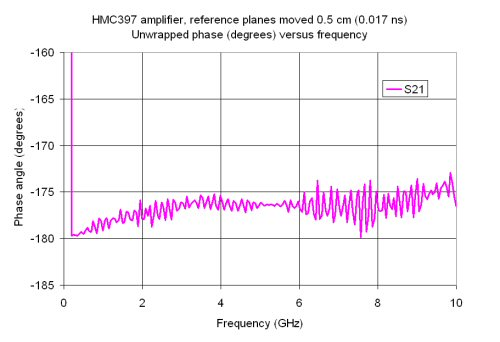Now when we look at group delay, we see that it is essentially zero, which verifies that we have moved the reference planes so that the DUT has zero length: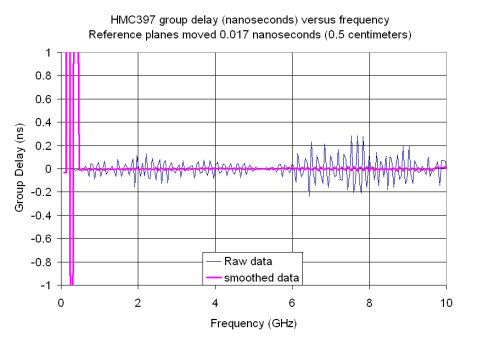The data that Hittite supplied was measure just about every 50 MHz. At this interval the phase only moves about 1.5 degrees between each point. It the data is only accurate to 0.1 degrees, this is the source of error that makes the group delay jumpy.

It's time for another Microwaves101 rule of thumb!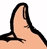When measuring S-parameters to get group delay, you should pick the frequency interval to achieve about 10 degrees S21 phase difference between frequency points. Less than this will make the measurement jumpy, greater than 10 degrees might mask some real problems in group delay flatness. How do you know in advance what frequency interval to pick? Excuse us while we go think up a formula for this...

That's a subject for another day!

Author : Unknown Editor